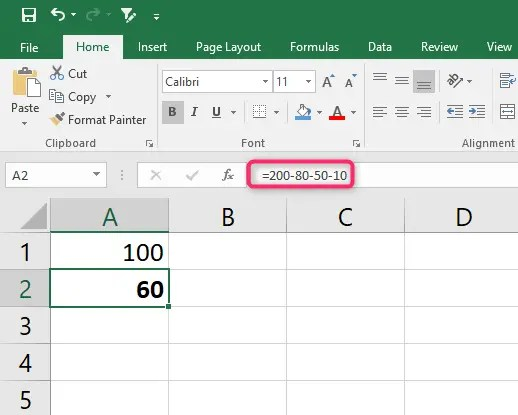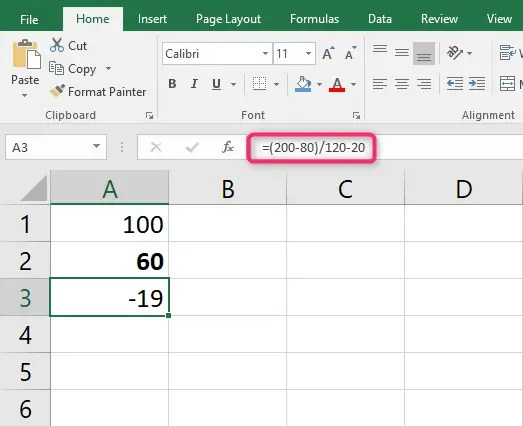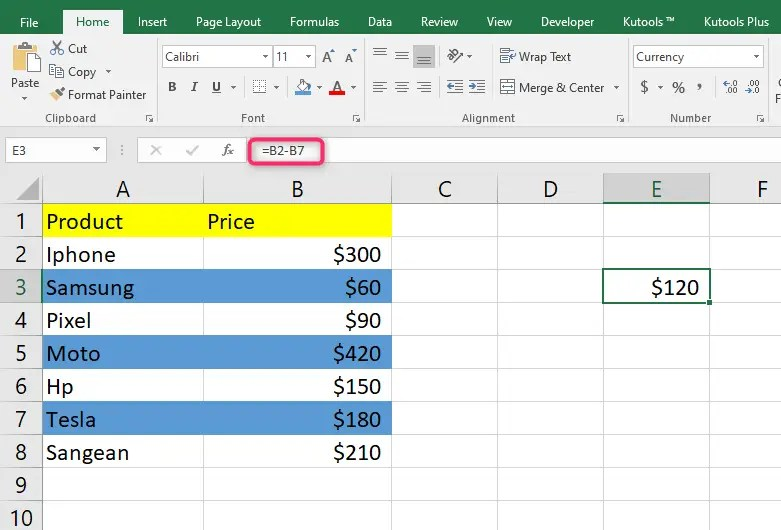# How to calculate in excel (minus)

The use of subtraction is a popular method of calculating using a minus sign. Since the primary level, subtraction has been used regularly, and many are familiar. Apparently, this method also works in Excel. You can use it to subtract a number of things, including percentages, minutes, numbers, hours, seconds, days, and more. Moreover, excel can even subtract text strings, matrices, and lists. Therefore, having knowledge of minus in excel can be very useful. Without further ado, here are ways how to calculate in Excel (minus).

• Excel subtraction formula
• Subtraction cells in Excel
• Subtract columns

Those are the ways you can use to subtract in Excel. Let’s get into the details of the above methods. We have also included the steps of the methods and screenshots of the above methods.

## Excel subtraction formula ( minus) formula

Excel doesn’t have the SUBTRACT function; however, you can use a minus sign to perform a simple subtraction. So, let me take you through a simple.

If you are given two numbers, that’s number 1 and number 2. We can use 100 and 50. You want to calculate with a minus.

That’s

Number 1- number 2

= 100-50

Follow the following steps to use the Excel subtraction formula (minus) formula to calculate.

Steps

1. Go to the cell where you want the results to appear and type the Equal sign. (=)

2. In the cell, type number 1 minus sign, then number 2.

3. Press the “Enter” key to complete.In math, you can minus several numbers by using a single formula. For instance, you can subtract a few numbers from 200. You can type the formula as shown below.

=200-80-50-10You can use parentheses to indicate which formula part you want to subtract first. For example, as shown below.

=(200-80)/120-20)## Subtract cells in Excel (procedures)

You can also use the minus formula to subtract cells in your worksheet. However, you’ll use cell references instead of actual numbers. Check example below

=Cell_1 – Cell_2

For instance, to subtract values in cell B2 from A2, you can use the following formula.

=A2 – B2

You can subtract without necessarily typing the values from the cells. Just take their corresponding cells to subtract the values. Check the steps below;

Steps

2. Click on the cell that has the number, which you’ll subtract the other from(minuend number). It will be added to your formula automatically,

3. Type the minus (-) sign.

4. Click on the cell which has the number to be subtracted (subtrahend) it’s reference will be added to the formula

5. Hit the “enter” key to complete the formula.

6. You’ll then have your results like the one shown belowThe above are the steps to subtract using single cells in Excel. You can also subtract multiple cells in Excel using the following methods.

• Minus sign
• Sum function
• Sum negative numbers